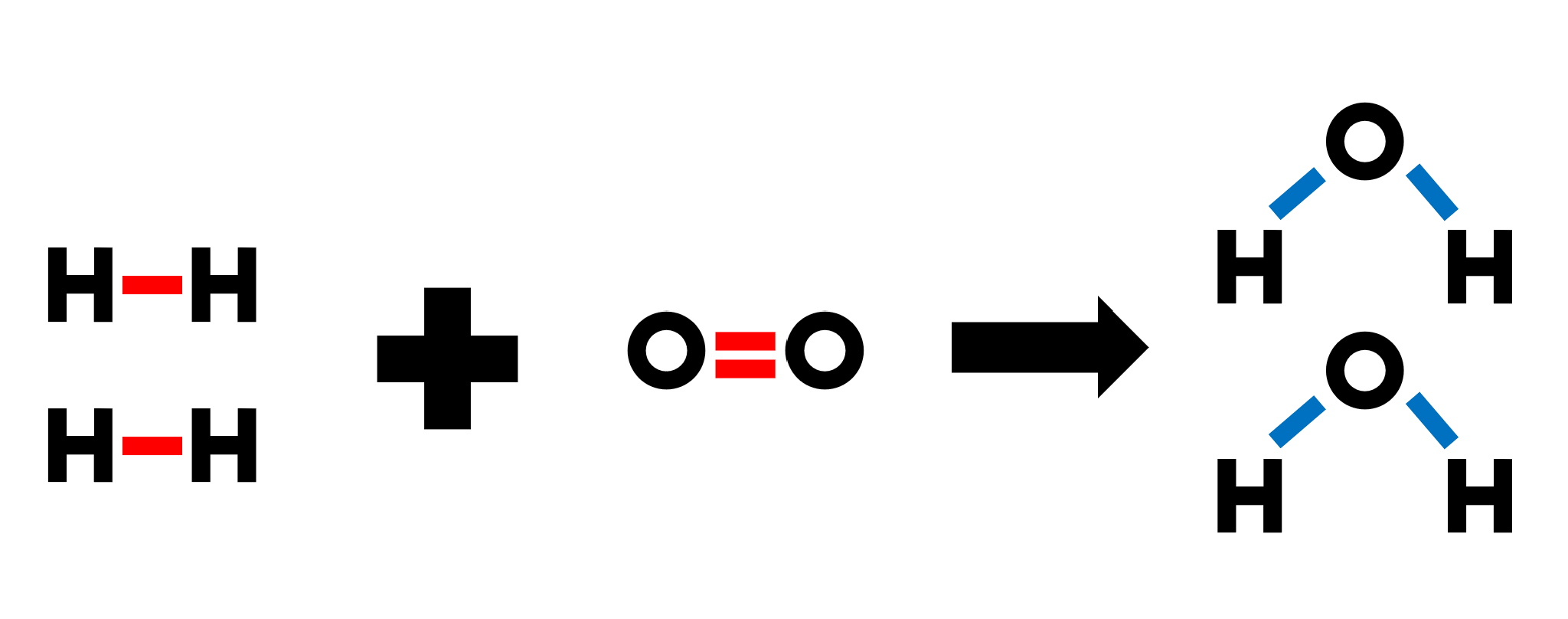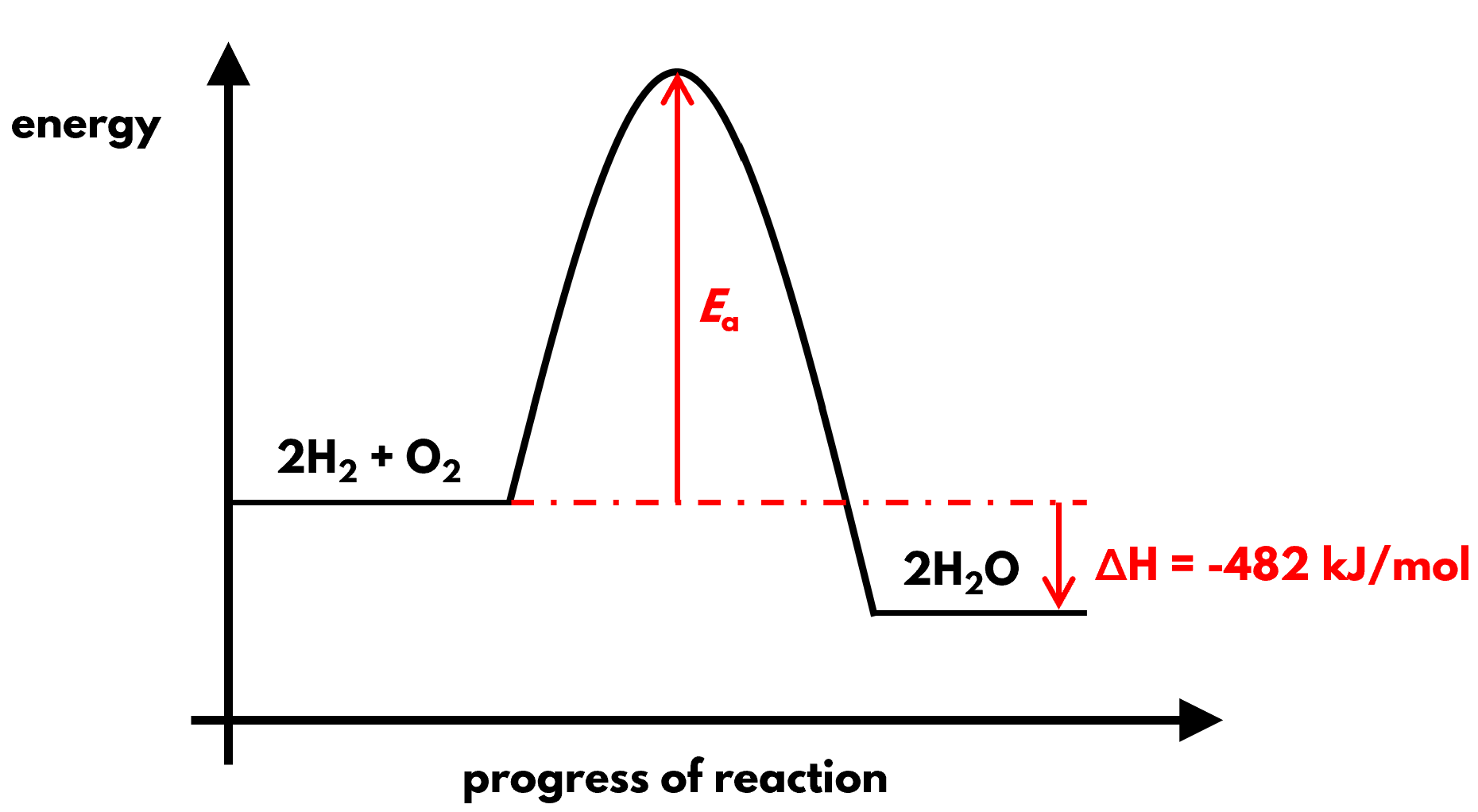## 1. Exothermic reactions turn up the heat and power our lives

© Prince Photos on Pexels.com

In the morning, gentle flame caresses kettles of boiling water to awake the coffee beans and tea leaves that in turn awake our senses. Later in the evening, gas stoves roar to fry up plates of mee goreng and the like. While this took different forms in the past, people since time immemorial have been burning chemicals to release energy for cooking and warmth. We call such reactions that give out heat exothermic reactions.

We call the gain or loss of energy during a reaction enthalpy change, ΔH. Because enthalpy change is a measurement of energy, it can be given in the SI unit joules, J. However, it is more commonly given in kilojoules, kJ or kilojoules per mole of a substance, kJ/mol.

H2(g) + O2(g) ⟶ H2O(l), ΔH = -286 kJ/mol

Exothermic reactions have negative enthalpy change. The negative sign signifies the loss of energy when reactants are transformed into products. For example, the exothermic combustion of 1 mol of methane gas has an enthalpy of -286 kJ. This means that 286 kJ of heat is lost to the surrounding. Besides combustion, other exothermic reactions include:

• Neutralisation
• Physical changes like condensation and freezing

## 2. The opposite: chill out with endothermic reactions

Other reactions absorb heat energy from the surrounding, causing a concomitant decrease in temperature. What a relief they would provide in tropical Singapore! We call these shiok reactions endothermic reactions.

H2O(s) ⟶ H2O(l), ΔH = +6 kJ/mol

For example, when we eat bingsu and the ice melts in our mouth, we feel an instant chill. This is because melting is an endothermic reaction. Heat is absorbed from our body to overcome the intermolecular forces of attraction holding the water molecules together in ice. This is reflected by the enthalpy of +6 kJ/mol, whereby the positive sign reflects the heat gained by the chemical system.

## 3. The tug of war between bond breaking and bond making

So why is the combustion of hydrogen exothermic but melting endothermic? One way to rationalise it is to consider the molecular processes during a reaction: bond forming and bond breaking.

• Bond breaking requires energy, and is hence endothermic
• Bond forming releases energy, and is hence exothermic

Melting is endothermic because it only involves bond breaking but not bond forming. However, explaining why combustion is exothermic is not as straightforward. We have to consider both processes to see which involves a greater energy change.Overall, the red bonds are broken while the blue bonds are formed during the combustion of hydrogen.

While the actual sequence of bond breaking and forming may be very complicated, we shall look at the overall changes. On the whole, 2 H-H single bonds and 1 O=O double bond are broken, while 4 O-H bonds are formed. The reaction is exothermic because more energy is released during bond forming than that absorbed during bond breaking. We can show this by means of a calculation.

## 4. How to calculate enthalpy change from bond energy

We can calculate the total enthalpy change of a reaction based on the energy required to break bonds and released to form bonds. These data are given in the form of bond energy.

The value of bond energy is always positive, as it reflects the energy required for bond breaking, an endothermic process. To use the data for exothermic bond forming, we have to make it negative. As such, the formula to calculate the enthalpy change of a reaction is:

enthalpy change, ΔH = bond energy of bonds broken – bond energy of bonds formed

We shall apply this formula to calculate the enthalpy change of combustion of hydrogen.

• ### STEP 1: Find the total bond energy of bonds broken

Energy required to break 2 H-H = 2× 436 = 872 kJ/mol
Energy required to break 1 O=O = 498 kJ/mol
Total energy required for bond breaking = 872 + 498 = 1370 kJ/mol

• ### STEP 2: Find the total bond energy of bonds formed

Energy released on forming 4 O-H = 4 × 463 = 1852 kJ/mol

If your statement is “energy change” instead of “energy released”, you will have to write your answer as “-1852 kJ/mol”. The negative sign must be included to show that the energy is lost by the chemicals.

• ### STEP 3: Apply the formula to calculate enthalpy change

Enthalpy change = 1370 – 1852 = -482 kJ/mol

Don’t forget the negative sign to show that the enthalpy change is exothermic, where energy is lost to the surrounding.

## 5. Activation energy: the threshold to react

Wait a minute! If exothermic reactions like combustion does not require energy but releases energy, won’t everything burn up randomly and perpetually? But things don’t.

This is because all reactions involve activation energy, Ea. It is the energy that sleepy reactants must first absorb to become activated for the reaction to proceed. In other words, activation energy is an energy barrier that prevents things from burning randomly.

## 6. How to draw an energy profile diagram

We can represent both enthalpy change and activation energy using a diagram that we call the energy profile diagram.Energy profile diagram for the combustion of hydrogen, which is typical of an exothermic reaction

In the energy profile diagram showing the combustion of hydrogen, the energy level of the product is lower than that of the reactants. This is typical of an exothermic reaction. Due to the loss of energy, the products have less energy after the reaction.Energy profile diagram for the melting of ice, which is typical of an endothermic reaction

For endothermic reactions like the melting of ice, their energy profile diagram likewise have a hump representing the activation energy. However, the energy level of the product is higher, as energy has been gained during the reaction.

For the O Level, your energy profile diagram should show:

• Reactants and products
• Activation energy, Ea
• The exact value of the enthalpy change, ΔH